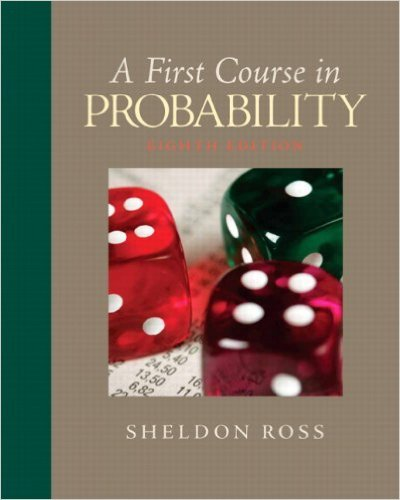×
×

# n and m are positive numbers.(a) If two balls are randomlyISBN: 9780136033134 163

## Solution for problem 29 Chapter 2

First Course in Probability | 8th Edition

• Textbook Solutions
• 2901 Step-by-step solutions solved by professors and subject experts
• Get 24/7 help from StudySoup virtual teaching assistantsFirst Course in Probability | 8th Edition

4 5 1 269 Reviews
25
0
Problem 29

n and m are positive numbers.(a) If two balls are randomly withdrawn, what isthe probability that they are the same color?(b) If a ball is randomly withdrawn and thenreplaced before the second one is drawn, whatis the probability that the withdrawn balls arethe same color?(c) Show that the probability in part (b) is alwayslarger than the one in part (a).

Step-by-Step Solution:
Step 1 of 3

X, Y Bivariate Random Variables f(u1, 2 ) is density of (X,Y) ∫ ( ) density of X ∫ ( ) density of Y X, Y are independent if f(u1, 2 = f1(u1 2 (u2) If X1, ,2…….X are nndependent Var(X 1 ……..X ) = n + …….. We want to produce to specification “m” “m” = length of a part, content of juice can etc We want to check at the...

Step 2 of 3

Step 3 of 3

##### ISBN: 9780136033134

Unlock Textbook Solution